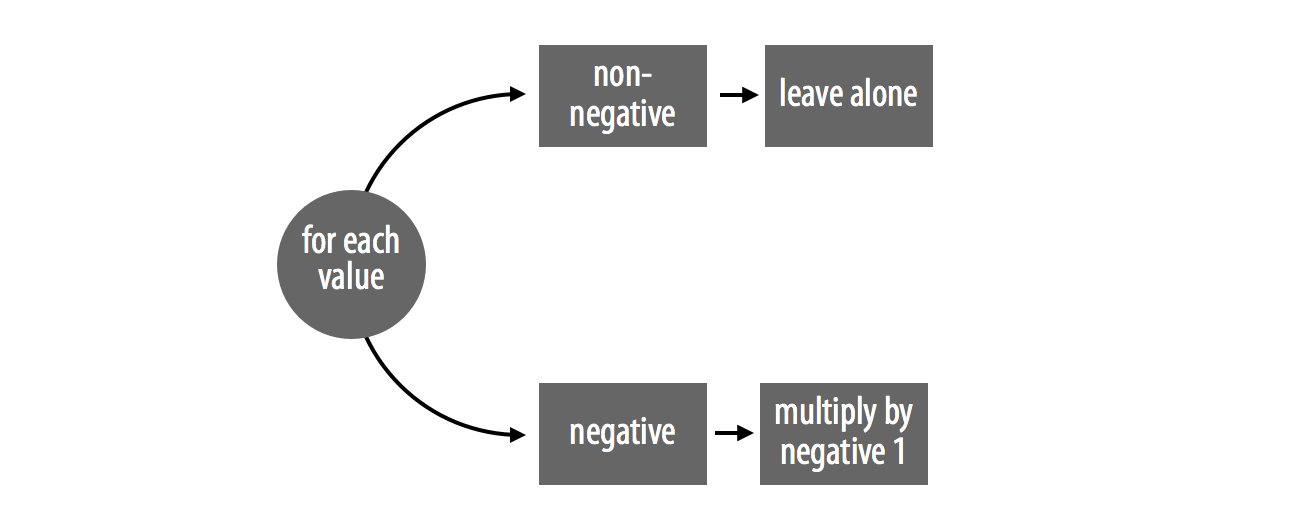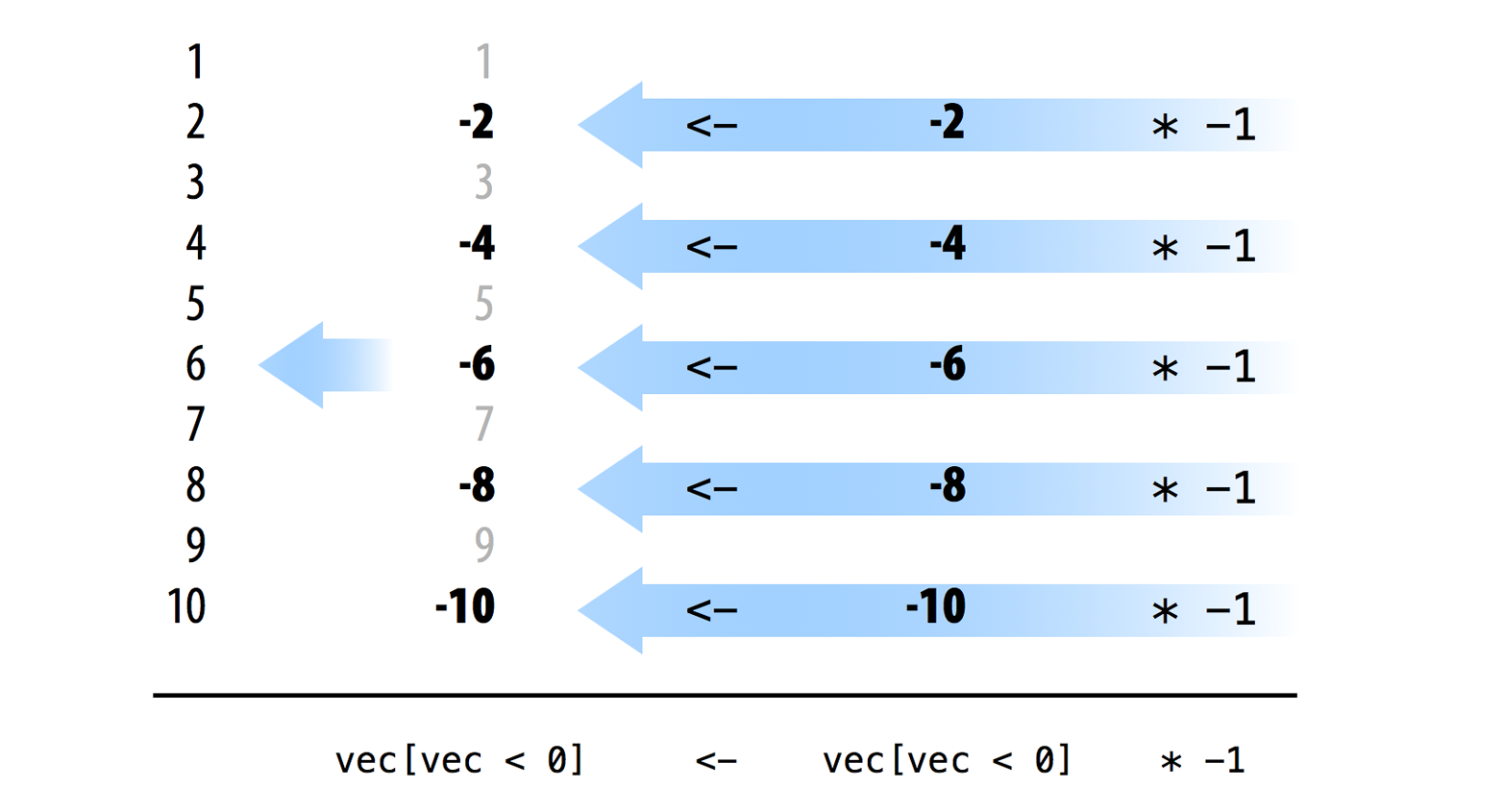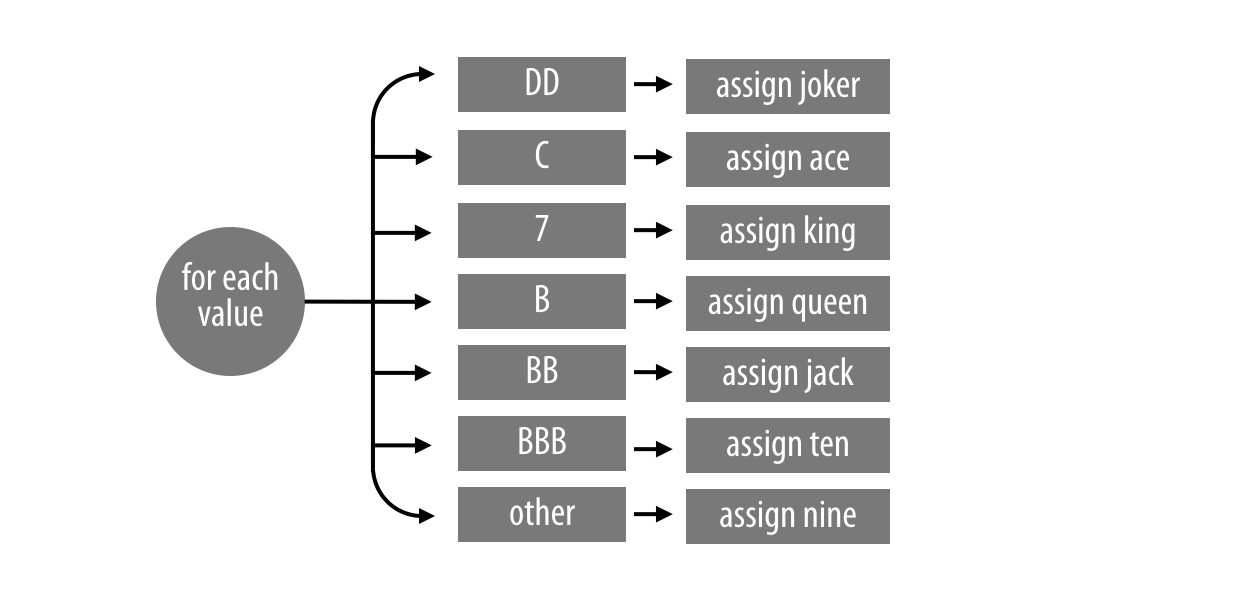## Vectorized Code

• The fastest R code will usually take advantage of three things:
• logical tests
• subsetting
• element-wise execution
• Code that uses these things usually has a certain quality: it is vectorized.
• That means the code can take a vector of values as input and manipulate each value in the vector at the same time (parallel computing).

## Vectorized Code

• Example: Write an absolute value function in R that takes
• Input: vec a vector of numbers
• Ouput: a vector with all non-negative numbers

## Vectorized Code

• First method: for loop
abs_loop <- function(vec){
for (i in 1:length(vec)) {
if (vec[i] < 0) {
vec[i] <- -vec[i]
}
}
vec
}

## Vectorized Code

• Second method: vectorized code
abs_vectorized <- function(vec){
negs <- vec < 0
vec[negs] <- vec[negs] * -1
vec
}

## Vectorized Code

• Claim: abs_vectorized() is much faster than abs_loop().
• Let test this claim!
long <- rep(c(-1, 1), 5000000)

system.time(abs_loop(long))
##    user  system elapsed
##   0.983   0.045   1.051
system.time(abs_vectorized(long))
##    user  system elapsed
##   0.261   0.065   0.336

## Vectorized Code

• How does our abs_vectorized() function compare to abs() built-in function?
system.time(abs(long))
##    user  system elapsed
##   0.023   0.000   0.024

## How to Write Vectorized Code

• To create vectorized code:
• Use vectorized functions (R built-in functions) to complete the sequential steps in your program.
• Use logical subsetting to handle parallel cases. Try to manipulate every element in a case at once.

## Example: abs_vectorized()## How to Write Vectorized Code

vec <- c(1, -2, 3, -4, 5, -6, 7, -8, 9, -10)
vec < 0
##   FALSE  TRUE FALSE  TRUE FALSE  TRUE FALSE  TRUE FALSE  TRUE
vec[vec < 0]
##   -2  -4  -6  -8 -10
vec[vec < 0] * -1
##   2  4  6  8 10
vec
##     1  -2   3  -4   5  -6   7  -8   9 -10

## How to Write Vectorized Code## Exercise 1

change_symbols <- function(vec){
for (i in 1:length(vec)){
if (vec[i] == "DD") {
vec[i] <- "joker"
} else if (vec[i] == "C") {
vec[i] <- "ace"
} else if (vec[i] == "7") {
vec[i] <- "king"
}else if (vec[i] == "B") {
vec[i] <- "queen"
} else if (vec[i] == "BB") {
vec[i] <- "jack"
} else if (vec[i] == "BBB") {
vec[i] <- "ten"
} else {
vec[i] <- "nine"
}
}
vec
}

## Exercise 1

• The function shown converts a vector of slot symbols to a vector of new slot symbols.
• Can you vectorize it?
vec <- c("DD", "C", "7", "B", "BB", "BBB", "0")

change_symbols(vec)
##  "joker" "ace"   "king"  "queen" "jack"  "ten"   "nine"

## Exercise 1## Lookup Table

Another way to vectorize code is to use lookup table. Think lookup table as a dictionary.

change_vec2 <- function(vec){
tb <- c("DD" = "joker", "C" = "ace", "7" = "king", "B" = "queen",
"BB" = "jack", "BBB" = "ten", "0" = "nine")
unname(tb[vec])
}

## How to Write Fast for Loops in R

system.time({
output <- c()

for (i in 1:1000000) {
output[i] <- i + 1
}
})
##    user  system elapsed
##   0.372   0.097   0.479

## How to Write Fast for Loops in R

system.time({
output <- rep(NA, 1000000)

for (i in 1:1000000) {
output[i] <- i + 1
}
})
##    user  system elapsed
##   0.122   0.005   0.135

## Vectorized Code in Practice

• Remember Exercise 2 from Monday’s lecture?
• From a given list of fruits, select only the ones that have 6 or fewer letters.

## To-do

• Submit Homework 2. Due at 11:59 PM on Compass.
• Homework 3 will be published this afternoon.